# Domain Analysis Testing

Standing in the concessions car of the Orient Express as it hissed and lurched away from the station, Special Agent Chu could feel enemy eyes watching him from the inky shadows and knew that he was being tested, for although he had never tasted a plug of tobacco in his life, he was impersonating an arms dealer known to be a connoisseur, so he knew that he, the Chosen One, Chow Chu, had no choice but to choose the choicest chew on the choo-choo.

— Loren Haarsma

## Introduction

In the chapters on Equivalence Class and Boundary Value testing, we considered the testing of individual variables that took on values within specified ranges. In this chapter we will consider the testing of multiple variables simultaneously. There are two reasons to consider this:

• We rarely will have time to create test cases for every variable in our systems. There are simply too many.
• Often variables interact. The value of one variable constrains the acceptable values of another. In this case, certain defects cannot be discovered by testing them individually.

Domain analysis is a technique that can be used to identify efficient and effective test cases when multiple variables can or should be tested together. It builds on and generalizes equivalence class and boundary value testing to n simultaneous dimensions. Like those techniques, we are searching for situations where the boundary has been defined or implemented incorrectly.

 Key Point Domain analysis is a technique that can be used to identify efficient and effective test cases when multiple variables should be tested together.

In two dimensions (with two interacting parameters) the following defects can occur:

• A shifted boundary in which the boundary is displaced vertically or horizontally
• A tilted boundary in which the boundary is rotated at an incorrect angle
• A missing boundary
• An extra boundary

Figure 8-1 is adapted from Binder. It illustrates these four types of defects graphically.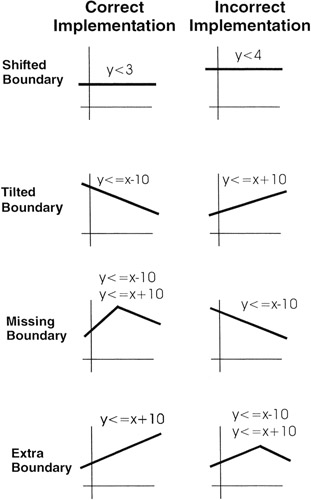Figure 8-1: Two dimensional boundary defects.

Certainly there can be interactions between three or more variables, but the diagrams are more difficult to visualize.

## Technique

The domain analysis process guides us in choosing efficient and effective test cases. First, a number of definitions:

• An on point is a value that lies on a boundary.
• An off point is a value that does not lie on a boundary.
• An in point is a value that satisfies all the boundary conditions but does not lie on a boundary.
• An out point is a value that does not satisfy any boundary condition.

Choosing on and off points is more complicated that it may appear.

• When the boundary is closed (defined by an operator containing an equality, i.e., ≤, ≥ or =) so that points on the boundary are included in the domain, then an on point lies on the boundary and is included within the domain. An off point lies outside the domain.
• When the boundary is open (defined by an inequality operator < or >) so that points on the boundary are not included in the domain, then an on point lies on the boundary but is not included within the domain. An off point lies inside the domain.

Confused? At this point examples are certainly in order.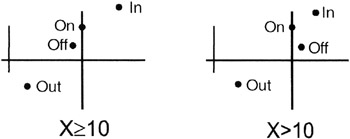Figure 8-2: Examples of on, off, in, and out points for both closed and open boundaries.

On the left is an example of a closed boundary. The region defined consists of all the points greater than or equal to 10. The on point has the value 10. The off point is slightly off the boundary and outside the domain. The in point is within the domain. The out point is outside the domain.

On the right is an example of an open boundary. The region defined consists of all the points greater than (but not equal to) 10. Again, the on point has a value of 10. The off point is slightly off the boundary and inside the domain. The in point is within the domain. The out point is outside the domain.

Having defined these points, the 1x1 ("one-by-one") domain analysis technique instructs us to choose these test cases:

• For each relational condition (, >, , or <) choose one on point and one off point.
• For each strict equality condition (=) choose one on point and two off points, one slightly less than the conditional value and one slightly greater than the value.

Note that there is no reason to repeat identical tests for adjacent domains. If an off point for one domain is the in point for another, do not duplicate these tests.

Binder suggests a very useful table for documenting 1x1 domain analysis test cases called the Domain Test Matrix.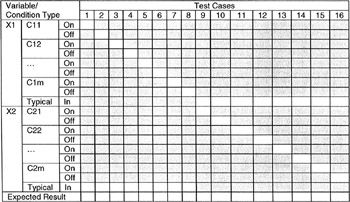Note that test cases 1 through 8 test the on points and off points for each condition of the first variable (X1) while holding the value of the second variable (X2) at a typical in point. Test cases 9 through 16 hold the first variable at a typical in point while testing the on and off points for each condition of the second variable. Additional variables and conditions would follow the same pattern.

## Example

Admission to Stateless University is made by considering a combination of high school grades and ACT test scores. The shaded cells in the following table indicate the combinations that would guarantee acceptance. Grade Point Averages (GPAs) are shown across the top while ACT scores are shown down the left side. Stateless University is a fairly exclusive school in terms of its admission policy.

Explanation

The ACT Assessment is an examination designed to assess high school students' general educational development and their ability to complete college-level work.

The Grade Point Average is based on converting letter grades to numeric values

A = 4.0 (Best)

B = 3.0

C = 2.0 (Average)

D = 1.0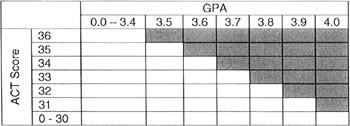This table can be represented as the solution set of these three linear equations:

ACT ≤ 36 (the highest score possible)

GPA ≤ 4.0 (the highest value possible)

10*GPA + ACT ≥ 71

(The third equation can be found by using the good old y=mx+b formula from elementary algebra. Use points {ACT=36, GPA=3.5} and {ACT=31, GPA=4.0} and crank—that's math slang for solve the pair of simultaneous equations obtained by substituting each of these two points into the y=mx+b equation.)Figure 8-3: Stateless University Admissions Matrix in graphical form.

The following test cases cover these three boundaries using the 1x1 domain analysis process.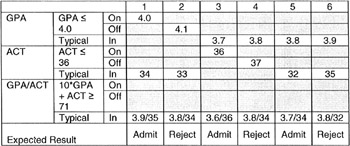Test cases 1 and 2 verify the GPA ≤ 4.0 constraint. Case 1 checks on the GPA = 4.0 boundary while case 2 checks just outside the boundary with GPA = 4.1. Both of these cases use typical values for the ACT and GPA/ACT constraints.

Test cases 3 and 4 verify the ACT ≤ 36 constraint. Case 3 checks on the ACT = 36 boundary while case 4 checks just outside the boundary with ACT = 37. Both of these cases use typical values for the GPA and GPA/ACT constraints.

Test cases 5 and 6 verify the 10*GPA + ACT ≥ 71 constraint. Case 5 checks on the GPA = 3.7 and ACT = 34 boundary while case 6 checks just outside the boundary with GPA=3.8 and ACT = 32. Both of these cases use typical values for the GPA and ACT constraints.

## Applicability and Limitations

Domain analysis is applicable when multiple variables (such as input fields) should be tested together either for efficiency or because of a logical interaction. While this technique is best suited to numeric values, it can be generalized to Booleans, strings, enumerations, etc.

## Summary

• Domain analysis facilitates the testing of multiple variables simultaneously. It is useful because we rarely will have time to create test cases for every variable in our systems. There are simply too many. In addition, often variables interact. When the value of one variable constrains the acceptable values of another, certain defects cannot be discovered by testing them individually.
• It builds on and generalizes equivalence class and boundary value testing to n simultaneous dimensions. Like those techniques, we are searching for situations where the boundary has been implemented incorrectly.
• In using the 1x1 domain analysis technique for each relational condition (≥, >, ≤, or <) we choose one on point and one off point. For each strict equality condition (=) we choose one on point and two off points, one slightly less than the conditional value and one slightly greater than the value.

## Practice

1. Stateless University prides itself in preparing not just educated students but good citizens of their nation. (That's what their advertising brochure says.) In addition to their major and minor coursework, Stateless U. requires each student to take (and pass) a number of General Education classes. These are:

• College Algebra (the student may either take the course or show competency through testing).
• Our Nation's Institutions—a survey course of our nation's history, government, and place in the world.
• From four to sixteen hours of Social Science courses (numbers 100–299).
• From four to sixteen hours of Physical Science courses (numbers 100–299)
• No more than twenty-four combined hours of Social Science and Physical Science courses may be counted toward graduation.

Apply 1 x 1 domain analysis to these requirements, derive the test cases, and use Binder's Domain Test Matrix to document them.

## References

Beizer, Boris (1990). Software Testing Techniques. Van Nostrand Reinhold.

Binder, Robert V. (2000). Testing Object-Oriented Systems: Models, Patterns, and Tools. Addison-Wesley.

Preface

Section I - Black Box Testing Techniques

Section II - White Box Testing Techniques

Section IV - Supporting Technologies

Section V - Some Final Thoughts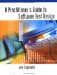A Practitioners Guide to Software Test Design
ISBN: 158053791X
EAN: 2147483647
Year: 2003
Pages: 161
Authors: Lee Copeland